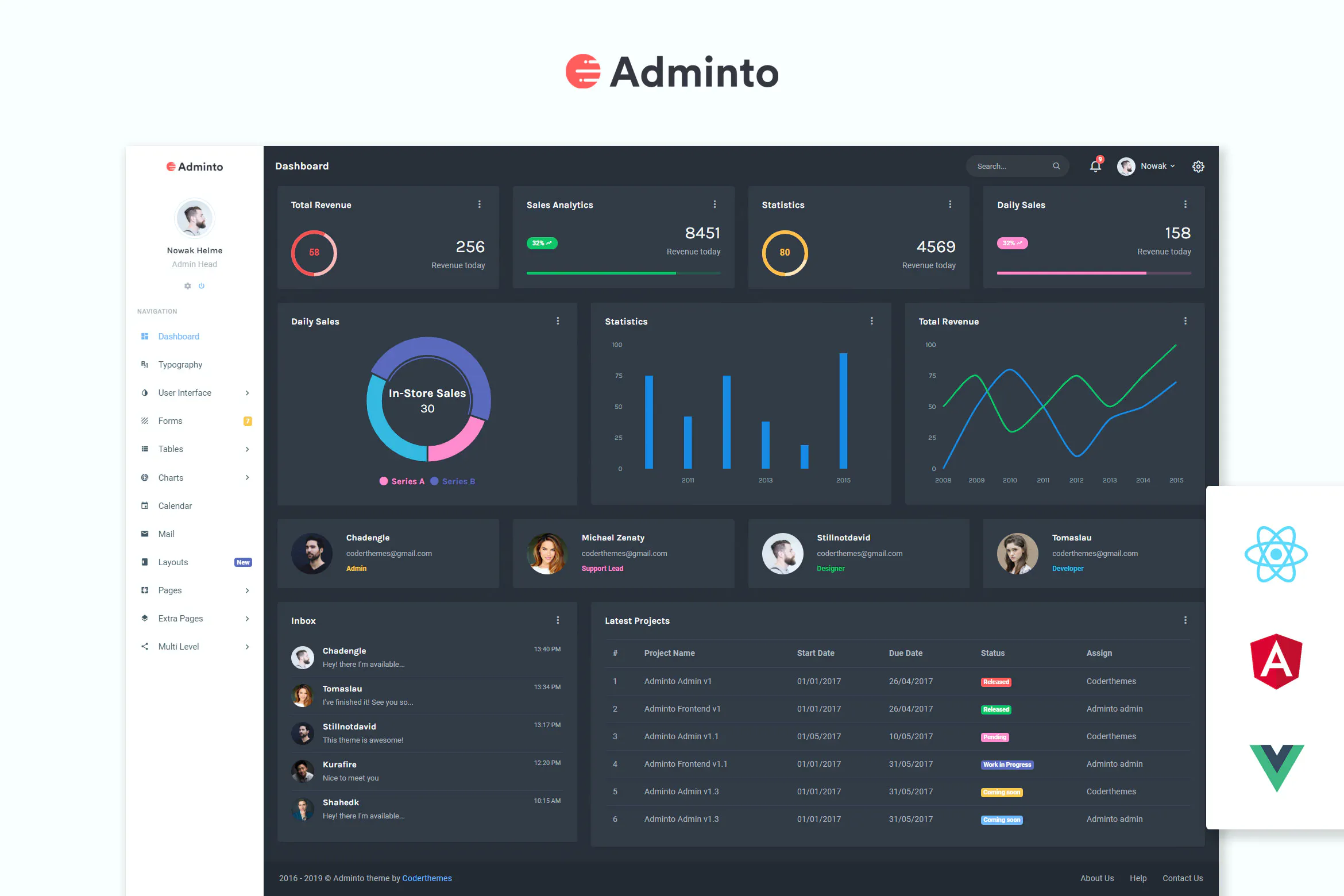• 完全响应式设计
• 光明和黑暗版本
• 使用Bootstrap v4.5.0创建
• 干净直观的设计
• 无限布局
• 完全记录
• 丰富的表单,小部件,验证,精灵
• 200 多页
• SASS支持 – Bootstrap 4 版本
• 包含错误页面
• RTL和LTR支持
• 包含登陆页面（一页）
• 时尚定价页面
• 4000+字体图标

Pages 文稿、应用程序：

• 仪表盘
• 排印
• 用户界面
• 按钮
• 卡片
• 可拖动卡片
• 复选框-收音机
• 材料设计图标
• 字体很棒
• 滴虫（新）
• 修改图标
• 模态
• 通知
• 范围滑块
• 部件
• 甜蜜警报
• 树景
• 小部件
• 表格
• 一般要素
• 高级表格
• 表单验证
• 表单向导
• 维西维格编辑
• 表单上传
• X-可编辑
• 桌子
• 基本表格
• 数据表
• 响应表
• 可编辑表
• 台锯台（新）
• 图表
• 情节图
• 莫里斯图表
• 图表
• Chartjs 2.0图表
• 其他图表
• 日历
• 电子邮件
• 页面
• 入门页面
• 登录
• 注册
• 恢复密码
• 锁定屏幕
• 确认邮件
• 错误404
• 错误500
• 其他
• 项目
• 旅行
• 任务板
• 任务详细信息
• 个人资料
• 地图
• 联系人列表
• 定价
• 时间轴
• 发票
• 常见问题解答
• 展馆
• 电子邮件模板
• 维护
• 即将发布